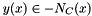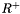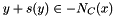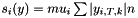So-Bogus A c++ sparse block matrix library aimed at Second Order cone problems
bogus::PyramidLaw< Dimension, Scalar, DeSaxceCOV > Class Template Reference

Experimental and incomplete pyramidal local solver that can be used within GaussSeidel and ProjectedGradient solvers. More...

#include <PyramidLaw.hpp>

## Public Types

enum  { dimension = Dimension }

typedef LocalProblemTraits
< Dimension, Scalar >
Traits

## Public Member Functions

PyramidLaw (const unsigned n, const double *mu)
Constructor. More...

Scalar eval (const unsigned problemIndex, const typename Traits::Vector &x, const typename Traits::Vector &y) const

bool solveLocal (const unsigned problemIndex, const typename Traits::Matrix &A, const typename Traits::Vector &b, typename Traits::Vector &x, const Scalar scaling) const
Solve forwith y(x) = Ax + b.

void projectOnConstraint (const unsigned problemIndex, typename Traits::Vector &x) const
Projects x on.

template<typename Segment >
void dualityCOV (const unsigned problemIndex, const Segment &y, typename Traits::Vector &s) const
Computes the change of variable s(y) so that (x, y+s(y)) obeys an associated law. More...

## Detailed Description

### template<DenseIndexType Dimension, typename Scalar, bool DeSaxceCOV> class bogus::PyramidLaw< Dimension, Scalar, DeSaxceCOV >

Experimental and incomplete pyramidal local solver that can be used within GaussSeidel and ProjectedGradient solvers.

Warning
solveLocal() is currently implemented in a highly inefficient way. This NSLaw may be used to compare the results with SOCLaw, not to compare performance.
projectOnConstraint() only handles dimensions 2 and 3

For demonstration purposes. Since blocks are 1x1, other libraries are probably more suited.

Template Parameters
 Scalar the scalar type Dimension the dimension of the blocks of the global matrix

## Constructor & Destructor Documentation

template<DenseIndexType Dimension, typename Scalar , bool DeSaxceCOV>
 bogus::PyramidLaw< Dimension, Scalar, DeSaxceCOV >::PyramidLaw ( const unsigned n, const double * mu )

Constructor.

Parameters
 n the size of the global problem ( number of contacts ) mu array containing the apertures of each second order cone ( friction coefficients )

## Member Function Documentation

template<DenseIndexType Dimension, typename Scalar , bool DeSaxceCOV>
template<typename Segment >
 void bogus::PyramidLaw< Dimension, Scalar, DeSaxceCOV >::dualityCOV ( const unsigned problemIndex, const Segment & y, typename Traits::Vector & s ) const

Computes the change of variable s(y) so that (x, y+s(y)) obeys an associated law.

ie. Here C = K_{mu}, and if

Template Parameters
 DeSaxceCOV is true,template<DenseIndexType Dimension, typename Scalar , bool DeSaxceCOV>
 Scalar bogus::PyramidLaw< Dimension, Scalar, DeSaxceCOV >::eval ( const unsigned problemIndex, const typename Traits::Vector & x, const typename Traits::Vector & y ) const
Returns, where fb is the SOC Fischer-Burmeister function

The documentation for this class was generated from the following file: